# Time reference curve

When you press the Setup button on newly activated Reference curve line, the following Reference curve setup window will open: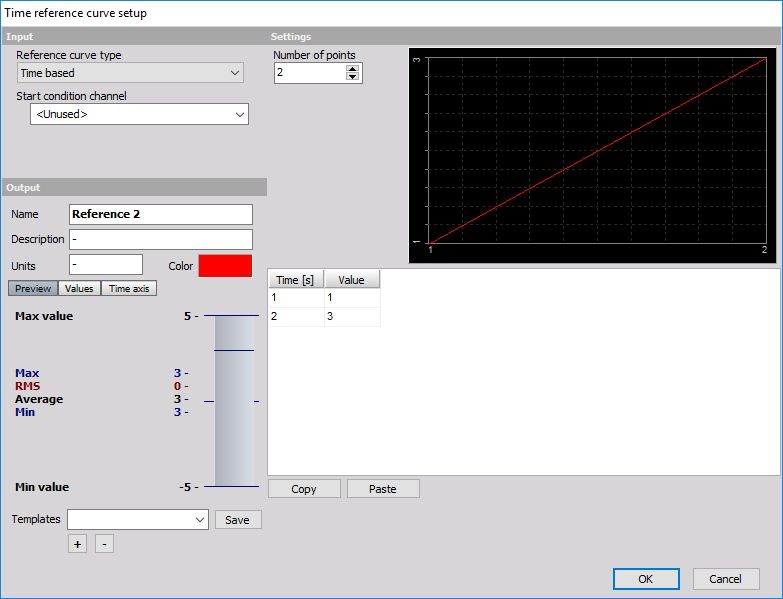## Input settings

### Reference curve type

In this field we can select Reference curve type from the drop-down list: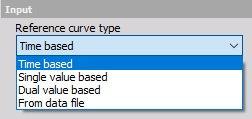#### Time based reference curve

Time based reference curve is useful for defining a curve in time as a reference during the certain test, which has to follow the certain protocol. Let’s say we have a test where we need to accelerate to 100 km/h in 10 seconds, drive constantly for 10 seconds and decelerate to 0 km/h in 10 seconds.

We can define for each curve starting criteria. If the Start condition channel is not defined, the curve will start at beginning of the measurement. Maybe it would be nice to define to start the test on a channel which measures vehicle velocity, but then we have also to define the limit Start if a value above (this field appear only when Start conditional channel is selected - default = \<Unused>). In our example we could enter for example 2 km/h.

Next step is to define Number of points and the points themselves in the list. In our case we would have four points, so we enter this in the field.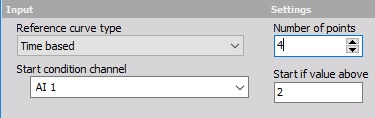A table will show four empty rows. Now we enter the time points and the values. In our case it will be: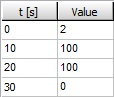• first point: 0s, 2 km/h
• second point: 10 s, 100 km/h
• third point: 20 s, 100 km/h
• fourth point: 30 s, 0 km/h

Display on right upper part Reference curve setup window now shows this curve: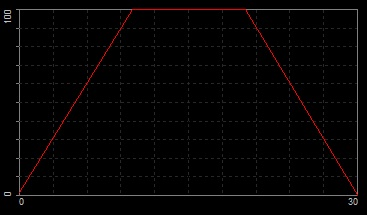Then we can use the formula math channel to see if we are in between or out of the limit. We can for example calculate:

$$\left|\text{RefCurve} - \text{Velocity}\right|>5$$

This will give a number of 1 if the absolute difference from reference curve to the measured signal is above 5 km/h and 0 if the difference is lower.

#### Single value based reference curve

A single value based reference curve can be understood as a sort of non-linear scaling. We need to define a First reference channel,

Number of points and a table with the values of reference and output channel.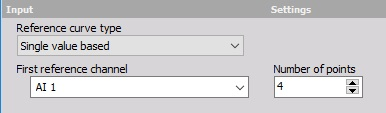So in this case on right, if the input channel will be 3, the output value will be 5. The values will be interpolated in between the points. So if the input is 2.5, the output will be 3.5. If the value is below or above the lowest or highest point, data will be extrapolated from two lowest or highest points. If we have 0 at the input, we will have 0 at the output. If we have 5 at the input, we will have 11 at the output.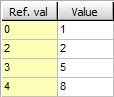Display on right upper part Reference curve setup window now shows this curve: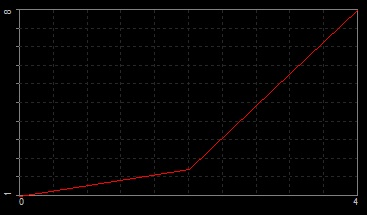#### Dual value based reference curve

Dual value based reference curve has two inputs: First reference channels and Second reference channels. We can imagine as the tridimensional surface of reference points. X and Y are two reference channels while the Z is defined points. We need to define first the channels and the Number of points for each channel.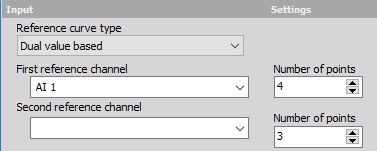Then we need to enter the reference values in the list.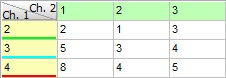Display on right upper part Reference curve setup window now shows this curve: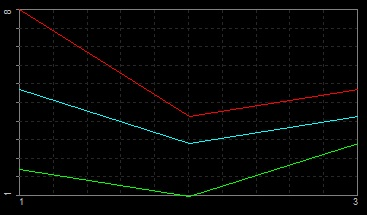A good example of using dual reference curve is to define the limit oil pressure referenced to RPM and the oil temperature.